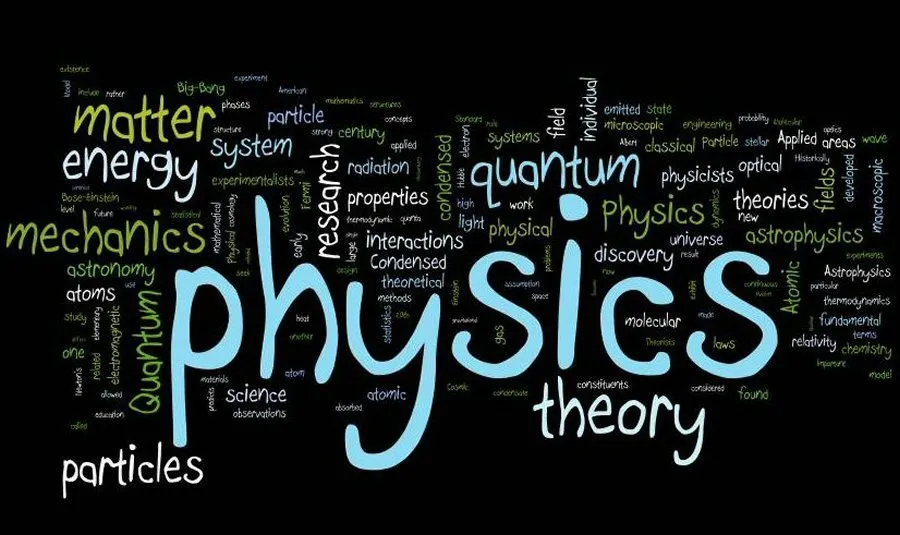# Physics - IntroductionHello it's a me again drifter1! Today we start with a new Category called Physics that will be all about Physics! This post is an general introduction to what physics is all about, models, measuring and so on! Later on we will get into each branch of physics one by one, starting of with Classical mechanics and Kinematics that is my favorite one :) So, without further do, let's get started!

## What is Physics?

Physics is a experimental science, which means that physicists observe the world around them and try to find rules and laws that apply around all the phenomena in the world. Those physics observations are called physics theories or even physics laws or rules if they are more general and find a better base to be defined.

So, a physicist must find the best questions, construct experiments and get results from the observations. This means that any general theory starts and ends with an experiment and can always be disproved if something new has been found! No theory is finallized!

## Models

Because the real world is complicated we construct models that are simplified versions of real systems.

Suppose we want to analyze the motion of a ball in the air. The ball is not a perfect sphere and also not  perfectly solid. The air and resistance of air affect the motion of the ball. The ball gets pushed down by gravity and the value of this gravity changes and depends on the distance of the ball to the center of the earth and on the earth's rotation. And we could find even more! If we used all these the analysis would become exceptionally difficult and so we find a more simple version of this system.

Let's neglect the size and shape of the ball and suppose that the ball is a simple point and all the mass is accumulated at the center of mass. Let's also discard that there is air and air resistance and suppose that we are in space and that there is vacuum. Let's also suppose that the accelaration of gravity is constant and doesn't depend on the earth's rotation or the distance of the ball to the center of the earth. Doing all that we now are in a much simpler problem that can easily be solved. That way the ball (point mass) now moves in a simple parabolic  path and we can use simple circular motion laws to find out the position, velocity and acceleration in time.

## Measurement:

Another important topic is how we measure. In physics we mostly use numbers to describe the results of the experiments. Each number that is used to describe a phenomenon is called a physics quantity. But, different physicists may use different standards. For example you all know that in the USA they use Fahrenheit and miles and in most european Countries we use Celsius and meters.

The system of measurement SI (Systeme International) is globally accepted and used by most scientists today.

When measuring we always have an error. The accurancy, important digits, estimation and order of magnitude are some important things we have to be cautious about!

## Vectors

Some measurements can not be described by the physics quantity and they value only. Those are the so called vector quantities. When moving from point A to B we have a value that describes how much we moved and also a vector that tells as to where we moved. The Displacement is a vector for example that describes how much we moved (meter of vector) and also the direction where we moved.

We will get into Vector Operations next time, cause there is a lot to cover!

And this is actually it and I hope that you enjoyed it!

Next time we will talk about Vectors!

Bye!

H2
H3
H4
3 columns
2 columns
1 column